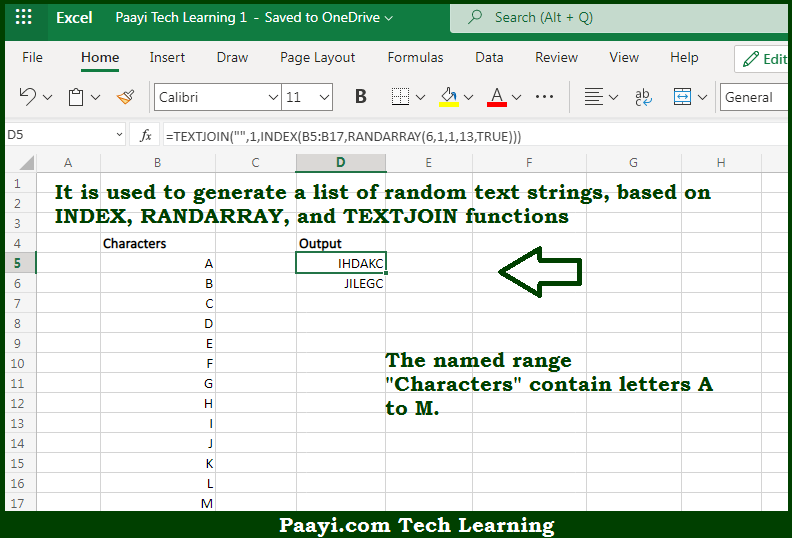# Learn How to Generate Random Text Strings in Microsoft Excel

Written by | 0 Comments | 632 Views

In this article, you will learn how to evaluate things in Dynamic Arrays with formulas in Microsoft Excel using a single/combination(s) of functions. You will also know How to Generate Random Text Strings and see the generic formula.

Learn How to Generate Random Text Strings in Microsoft Excel

The main purpose of this formula is to generate a list of random text strings. Here we will learn how to generate random text strings in the given data range in the workbook in Microsoft Excel. That implies, with the help of a formula based on the INDEX, RANDARRAY, and TEXTJOIN function you can able to generate a list of random text strings. So, with the help of this formula, you can able to generate random text strings in the given data range in the workbook in Microsoft Excel.

General Formula to Generate Random Text Strings

=INDEX(characters,RANDARRAY(n,1,1,count,TRUE))

The Explanation for the Generate Random Text StringsSo we know that with the help of the given formula above you can able to generate a list of random text strings. Here we will learn how to generate random text strings in the given data range in the workbook in Microsoft Excel. As we know that the new dynamic array formulas make it much easier to solve tricky problems with formulas.

Here, the goal is to generate a list of random 6-character codes. RANDARRAY function, handle the randomness of a new function. The results from the INDEX function are then concatenated together with the TEXTJOIN function. So, with the help of this formula, you can able to generate a list of random text strings. Here we will learn how to generate random text strings in the given data range in the workbook in Microsoft Excel.